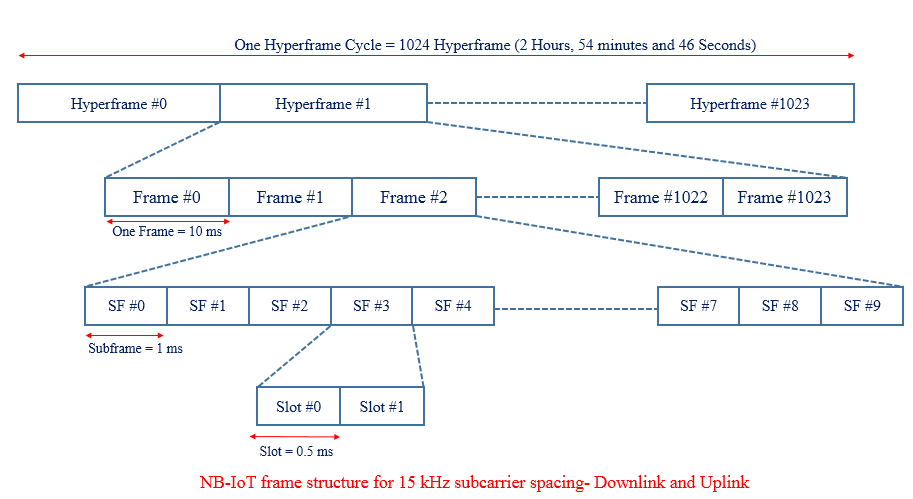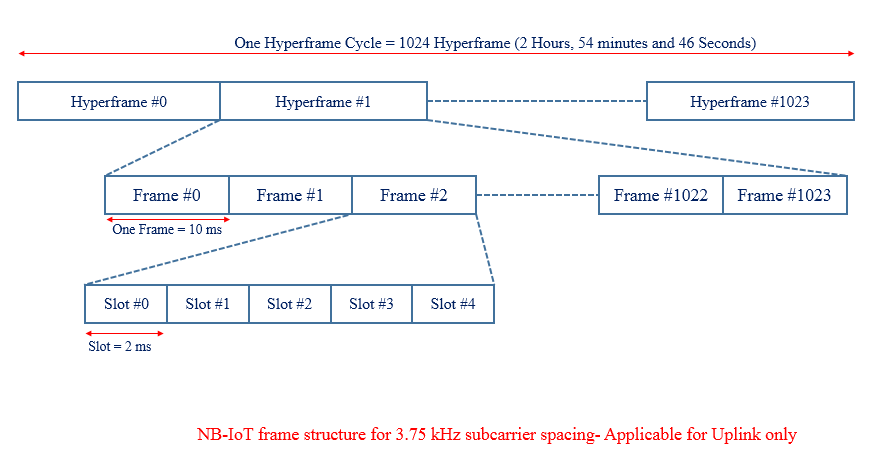# Narrow Band IoT Frame Structure

NB- IoT frame structure is illustrated in below picture.  At the highest level, it starts with the hyper frame cycle where one hyperframe cycle has 1024 hyper frames and each consisting of 1024 frames.

One frame consists of 10 sub-frames and each subframe is dividable into two slots each of 0.5 ms which is similar to the traditional LTE system. In the DL and UL, the NB-IoT design supports a sub-carrier spacing of 15 KHz, for which each frame contains 20 slots. In the UL, the NB-IoT design supports an additional sub-carrier spacing of 3.75 KHz. For this alternative sub-carrier spacing, each frame is directly divided into five slots, each of 2 ms, there is no subframe concept.

Case #1 Downlink and Uplink Subcarrier Spacing 15KHzCase #2 Uplink Subcarrier Spacing 3.75 KHzNotation and Range:

• Hyperframe:  Hyper System Frame Number (H-SFN), Range  : 0 – 1023
• Frame System Frame Number (SFN), Range  : 0 – 1023
• Subframe: Subframe Number (SN), Range  : 0 – 9

Each subframe can, therefore, be uniquely identified by an H-SFN, an SFN, and a subframe number (SN).

Cycle Duration:

• Duration of a Frame cycle =(No. of Frame in one cycle) x (Duration of one frame)                                                                                                  = 1024 x 10 ms =10240 ms = 10.24 seconds
• Duration of a Hyperframe cycle = (No. of Hyperframe in one cycle) x (Duration of one Hperframe)                                                                                 = 1024 x 10.24 s =104857.60 ms = 2 Hours, 54 minutes and 46 seconds
Recommended
The NPSS and NSSS help UE to synchronize downlink to…